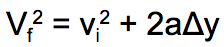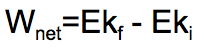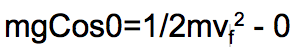Work-Energy Theorem applied to falling coin
1) Comparing a heavy coin with a light piece of chalk and ignoring the negligible air resistance...
2 points
2) The equations of motion...
2 points
3) Using the following formula and allowing a coin to fall for 1m, its velocity after falling 1 m (in m/s) is...
2 points4) The following formula can also be written...
2 points5) The Wnet done on a falling coin (projectile) is equal to...
2 points
6) The Work-Energy Theorem equation for a falling coin resolves into...
2 points
7) Because the Work-Energy Theorem for a falling object resolves into the following formula, the formula gives the same final velocity answer for any mass, because...
2 points